# 简单易懂的Dart基础语法，抽象类和接口。

#### 抽象类

``````abstractDemo() {
AbstractClass cls = AbstractClass();
}
abstract class AbstractClass{
}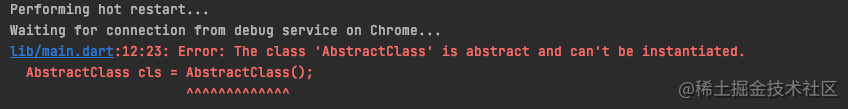``````abstract class AbstractClass{
// 这就是抽象方法
int sum(int a, int b);
}

``````class SubClass extends AbstractClass{
@override
int sum(int a, int b) {
print('a + b = \${a + b}');
return a + b;
}
}

abstractDemo() {
SubClass cls = SubClass();
cls.sum(10, 20);
}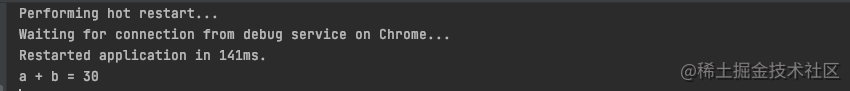#### 抽象类的实现

``````abstract class AbstractClass {
// 这就是抽象方法
int sum(int a, int b);
}

abstract class AbstractClass1 {
// 这就是抽象方法
int sum1(int a, int b);
}

abstract class AbstractClass2 {
// 这就是抽象方法
int sum2(int a, int b);
}

/// implements类似于接口
class SubClass implements AbstractClass, AbstractClass1, AbstractClass2 {
@override
int sum(int a, int b) {
print('a + b = \${a + b}');
return a + b;
}

@override
int sum1(int a, int b) {
print('a + b + 1 = \${a + b + 1}');
return a + b + 1;
}

@override
int sum2(int a, int b) {
print('a + b + 2 = \${a + b + 2}');
return a + b + 2;
}
}

abstractDemo() {
SubClass cls = SubClass();
cls.sum(10, 20);
cls.sum1(10, 20);
cls.sum2(10, 20);
}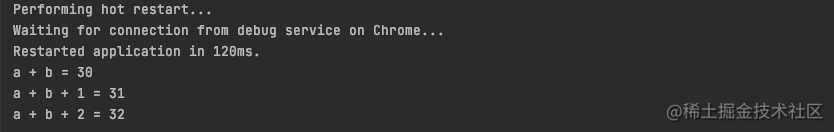#### Mixins混入

Mixins说白了就是多继承，D要多继承的话加一个`with`, D继承于A，BC是D的混入类。

``````class A {
a() => print('a...');
}

class B {
b() => print('b...');
}

class C {
c() => print('c...');
}

class D extends A with B, C {}

``````mixinDemo(){
D d = D();
d.a();
d.b();
d.c();
}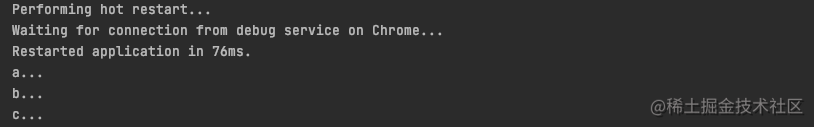``````class A {
a() => print('a...');
}

class B {
a() => print('b...');
}

class C {
a() => print('c...');
}

mixinDemo(){
D d = D();
d.a(); ///此时调用的a是哪一个
}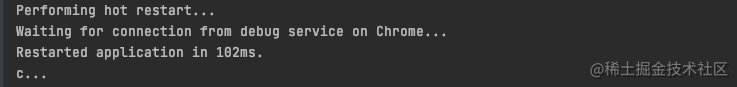``````class C {
C();
a() => print('c...');
}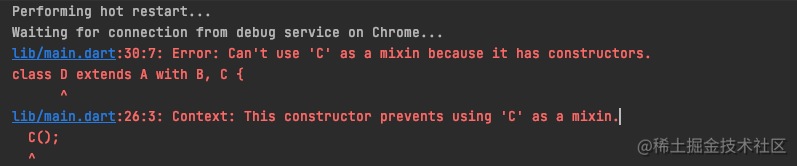``````class C extends TestClass {
a() => print('c...');
}

class TestClass {
}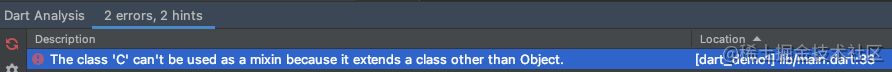``````// class D extends A with B, C {
// }
class D = A with B,C;

#### operator操作符

``````operatorDemo(){
OperatorClass ope1 = OperatorClass(10);
OperatorClass ope2 = OperatorClass(20);
print(ope1 > ope2); /// false
}

class OperatorClass{
int age;
OperatorClass(this.age); /// 构造函数
bool operator >(OperatorClass other) => this.age > other.age; /// 重载“>”操作符
}